Next: October 23 Up: October 18 Previous: October 18

Floyd-Warshall all-pairs shortest path

Here's an algorithm that can tolerate negative edges (but not negative cycles, even with only 2 vertices) and returns a table of the shortest distance between all pairs of vertices.

The idea is to first find all the minimal distances for pairs without using any intermediate vertices (this is easy to calculate, since it will be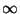if no edge exists between the pair, the edge weight otherwise). We then relax the restriction, step-by-step: first allow paths that use node 0 as an intermediate, and see whether the minimum distances can be adjusted downwards, then paths that are allowed to use nodes 0 and 1, and so on until all paths are considered. We denote the minimum distance from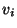to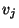that considers intermediate nodes from the set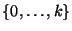as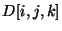(special case,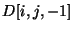doesn't allow any intermediate nodes).

Now we have a way of building up results. If we already know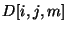, for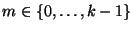, and every pair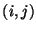, then the shortest path from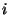to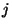either passes through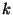or it doesn't. In the first case, the portion of this minimal path fromtois itself minimal and doesn't use(convince your self of this), as is the portion fromto, so=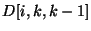+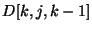. In the second case,equals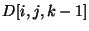.

Since we can easily calculatefor all pairs, we can build up to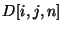(where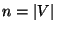) as follows (the third parameter is suppressed, but corresponds to the outer loop parameterin the bottom triply-nested loop):

FLOYD-WARSHALL( V, E )
for  i := 0  to  n-1  do
for  j := 0  to  n-1  do
if  i == j  then
D[i,j] := 0;
else if  (i,j) is in E  then
D[i,j] := w(i,j); /* <-- weight function w */
else
D[i,j] := oo;
end if
end for
end for
for  k := 0  to  n-1  do
for  i := 0  to  n-1  do
for  j := 0  to  n-1  do
if  D[i,k] + D[k,j] < D[i,j]  then
D[i,j] = D[i,k] + D[k,j];
end if
end for
end for
end for
END
Here's something to worry about. How do you know that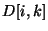and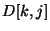correspond to,, and haven't been updated with minimum distances that pass through?

An easy calculation shows that Floyd-Warshall has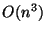complexity, which is consistent with using Dijkstra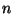times (and much simpler). How would you recover a shortest path, given this table of distances?Next: October 23 Up: October 18 Previous: October 18
Danny Heap 2002-12-16